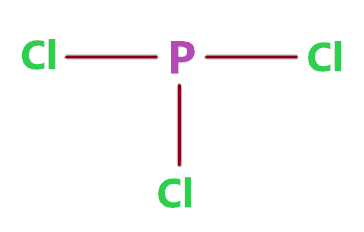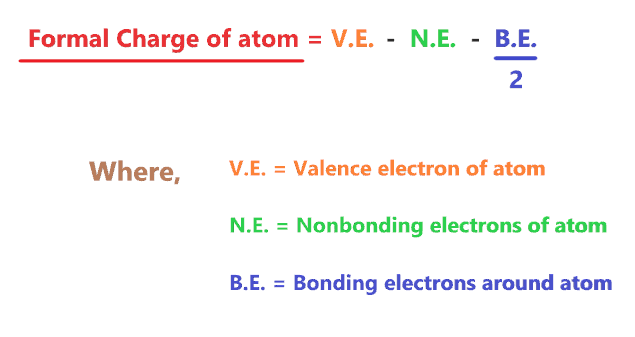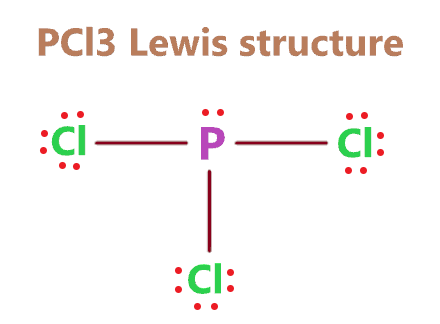# Lewis dot structure Calculator - Instant answer

Lewis structure calculator

A Lewis structure generator or calculator is an online tool that will help you to find the lewis structure for any atom or molecule. A Lewis structure is also known as the Lewis dot structure is a representation of electrons distribution around the atoms.

Lewis structure helps in determining the lone pair and bond pair in the molecule which is eventually helpful in predicting the shape or geometry of the molecule.

## How to use the Lewis structure calculator?

In three steps, you can get lewis’s structure for any atom or compound, let’s see how it works.

• Enter the “name” or “chemical formula” of the compound in the given blank box.
• Click the submit button
• After submission, a popup will appear and the lewis structure of the given molecule will be shown.

For example –

Let’s say you have to calculate the Lewis structure for ammonia (NH3).

⇒ Enter either the “Ammonia” or the “NH3” chemical formula in the given empty box of the Lewis structure calculator.⇒ Click the submit button⇒ The “Lewis structure generator” will compute the data and the lewis dot structure for ammonia will be shown in the Popup box.Check related Calculator Valence electron calculator Orbital diagram calculator Electron configuration calculator Formal charge calculator

As we know, Lewis structure helps in determining the lone pair and bond pair in the molecule which is eventually helpful in predicting the bond angle, shape or geometry of the molecule.

So, check this also

## How to Calculate Lewis structure?

For most of the compounds, the lewis structure is drawn using 5 or 6 simple steps-

1. Calculate the total number of valence electrons in the molecule
2. Choose the central and outer atoms
3. Draw a skeletal structure
4. Complete the octet of outer atoms first
5. Complete the octet of the central atom (make multiple bonds if necessary)
6. Check the formal charge for atoms

For example – Let’s say we have to calculate the lewis structure for the PCl3 molecule. For that, either you can use the online “Lewis structure calculator” tool or the traditional method.

Let’s follow some steps and draw the lewis structure for PCl3.

### Steps for drawing the Lewis dot structure for PCl3

1. Count total valence electron in PCl3

First of all, determine the valence electron that is available for drawing the lewis structure of PCl3 because the lewis diagram is all about the representation of valence electrons around atoms.

So, an easy way to find the valence electron of atoms in the PCl3 molecule is, just to look at the periodic group of phosphorous and chlorine atoms.

As the phosphorous atom belongs to the 5A group in the periodic table and chlorine is situated in the 7A group, hence, the valence electron for the phosphorous is 5, and for the chlorine atom, it is 7.

⇒ Total number of the valence electron in Phosphorous = 5

⇒ Total number of the valence electrons in chlorine = 7

∴ Total number of valence electron available for the PCl3 Lewis structure = 5 + 7×3 = 26 valence electrons         [∴ PCl3 molecule has one phosphorous and three chlorine atoms]2. Find the least electronegative atom and place it at center

An atom with a less electronegative value is preferable for the central position in the lewis diagram because they are more prone to share the electrons with surrounding atoms.

In the case of the PCl3 molecule, the phosphorous atom is less electronegative than the chlorine atom.

Hence, put the phosphorous atom at the central position of the lewis diagram and all three chlorine atoms outside to it.3. Connect outer atoms to the central atom with a single bond

In this step, join all outer atoms to the central atom with the help of a single bond.

In, the PCl3 molecule, chlorine is the outer atom, and phosphorous is the central atom. Hence, joined them as shown in the figure given below.Count the number of valence electrons used in the above structure. There are 3 single bonds used in the above structure, and one single bond means 2 electrons.

Hence, in the above structure, (3 × 2) = 6 valence electrons are used from a total of 26 valence electrons available for drawing the PCl3 Lewis structure.

∴ (26 – 6) = 20 valence electrons

So, we are left with 20 valence electrons more.

4. Place remaining electrons on the outer atom first and complete their octet

Let’s start putting the remaining valence electrons on outer atoms first. In the case of the PCl3 molecule, chlorine is the outer atom and each of them needs 8 electrons to have a full octet.

Start putting the remaining electrons on chlorine atoms as dots till they complete their octet.So, all chlorine atoms in the above structure completed their octet, because all of them have 8 electrons(6 electrons represented as dots + 2 electrons in every single bond) in their outer shell.

Now again count the valence electron in the above structure.

In the above structure, there is 18 electrons are represented as dots + three single bonds that contain 6 electrons means a total of 24 valence electrons is used in the above structure.

We have a total of 26 valence electrons available for drawing the lewis structure of PCl3. And we used 24 valence electrons in the above structure.

∴ (26 – 24) = 2 valence electrons

So, we are left with just 2 valence electrons.

5. Complete the octet of the central atom

Since we have already completed the octet for outer atoms in PCl3, now, check the octet of the central atom as well.

In PCl3, the central atom is phosphorous and it requires a total of 8 electrons to have a full valence shell.

If you look at the 4th step structure, the phosphorous atom is attached to three single bonds that means it have 6 electrons, so, it just short of 2 electrons.

We already have the remaining 2 valence electrons, hence, put these two electrons on the phosphorous atom to complete its octet as well.In the above structure, we see, that each atom completed its octet comfortably, now, Let’s check the formal charge for the above structure to verify whether it’s stable or not.

6. Check the stability with the help of a formal charge concept

The lesser the formal charge on atoms, the better the stability of the lewis diagram.

To calculate the formal charge on an atom. Use the formula given below-⇒ Formal charge = (valence electrons – nonbonding electrons –   1/2 bonding electrons)

Let’s count the formal charge for the 5th step structure.

For chlorine atom

⇒ Valence electrons of chlorine = 7

⇒ Nonbonding electrons on chlorine = 6

⇒ Bonding electrons around chlorine (1 single bond) = 2

∴ (7 – 6 – 2/2) = 0 formal charge on the chlorine atoms.

For phosphorous atom

⇒ Valence electrons of phosphorous = 5

⇒ Nonbonding electrons on phosphorous = 2

⇒ Bonding electrons around phosphorous (3 single bonds) = 6

∴ (5 – 2 – 6/2) = 0 formal charge on the phosphorous central atom.Hence, in the above PCl3 lewis structure, all atoms get a formal charge equal to zero.

Therefore, the above lewis dot structure of PCl3 (Phosphorous trichloride) is most stable and appropriate in nature.

That’s all by working on the methods given above, you can calculate the lewis structure for any compound.

Let’s check our full guide inHow to draw the lewis structure for any compound or ions?

Also, check the Lewis structure for some important molecules –

 CO2 Lewis structure N2H4 Lewis structure CH4 Lewis structure NH3 Lewis structure OF2 Lewis structure BrF5 Lewis structure CH2O Lewis structure CH3COOH Lewis structure BrO3- Lewis structure CHCl3 Lewis structure SO2Cl2 Lewis structure CN- Lewis structure BF3 Lewis structure PCl5 Lewis structure CH2Cl2 Lewis structure

## FAQ

Lewis structure or Lewis dot structure is drawn by using 5 or 6 steps.

• Calculate the total number of valence electrons in the molecule
• Choose the central and outer atoms
• Draw a skeletal structure
• Complete the octet of outer atoms first
• Complete the octet of the central atom (make multiple bonds if necessary)
• Check the formal charge for atoms

### How do you use the Lewis structure calculator tool?

It’s very simple, just put the name of the compound or chemical formula of the compound in the blank box of the “Lewis structure generator” tool, then, click on submit, and a popup box will appear with the Lewis structure of the given compound.

Did you like it?

#### Vishal Goyal

Vishal Goyal is the founder of Topblogtenz, a comprehensive resource for students seeking guidance and support in their chemistry studies. He holds a degree in B.Tech (Chemical Engineering) and has four years of experience as a chemistry tutor. The team at Topblogtenz includes experts like experienced researchers, professors, and educators, with the goal of making complex subjects like chemistry accessible and understandable for all. A passion for sharing knowledge and a love for chemistry and science drives the team behind the website. Let's connect through LinkedIn: https://www.linkedin.com/in/vishal-goyal-2926a122b/

Share it...

Topblogtenz is a website dedicated to providing informative and engaging content related to the field of chemistry and science. We aim to make complex subjects, like chemistry, approachable and enjoyable for everyone.